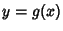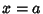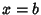## Solid of Revolution

To find the Volume of a solid of rotation by adding up a sequence of thin cylindrical shells, consider a region bounded above by, below by, on the left by the Line, and on the right by the Line. When the region is rotated about the y-Axis, the resulting Volume is given byTo find the volume of a solid of rotation by adding up a sequence of thin flat disks, consider a region bounded above by, below by, on the left by the Line, and on the right by the Line. When the region is rotated about the x-Axis, the resulting Volume is